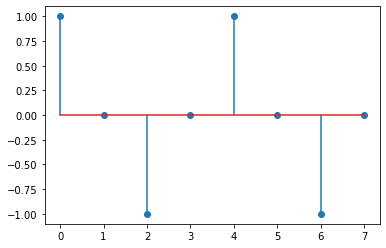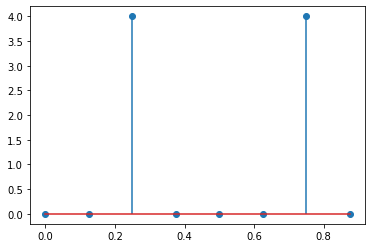In :
import numpy as np
import scipy as sp
import matplotlib.pyplot as plt

%matplotlib inline

In :
# A toy example shows how a sequence of samples from
# a time domain is mapped into a frequency domain using
# a Discrete Fourier Transformation.

k = np.arange(0,8)
x = np.cos(2*k*np.pi/4)

plt.stem(k, x, use_line_collection=True)

Out:
<StemContainer object of 3 artists>In :
X = sp.fft.fft(x)
print(X)

[-2.44929360e-16-0.00000000e+00j -3.46382422e-16+0.00000000e+00j
4.00000000e+00-9.79717439e-16j  3.46382422e-16+0.00000000e+00j
2.44929360e-16-0.00000000e+00j  3.46382422e-16-0.00000000e+00j
4.00000000e+00+9.79717439e-16j -3.46382422e-16-0.00000000e+00j]

In :
F = np.linspace(0, 1, len(X)+1)
F = F[:-1]

plt.stem(F, np.absolute(X), use_line_collection=True)

Out:
<StemContainer object of 3 artists>